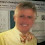## Sunday, February 14, 2016

### ∑{p≤ n} 1/p = ln(ln(n)) + o(1). read it here because....

(Last April fools day I  posted four links to stories that seemed absurd and asked which one was false. They all were true. I recently came across five  stories that all seem absurd but are all real, but three of them can't wait until April 1 since they are about current political events. Here they are:
Amazon to open many brick-and-mortar stores.
Why John Kasich got second place in New Hampshire (whch was better than expected).
Jim Gilmore's (who?) low expectations,
Why Ben Carson left Iowa.
airpnp- not about P vs NP
Donald Trump defends.... (I added this one on March 14)
And now back to our regularly scheduled blog)

A while back Larry Washington (number theorist at UMCP) showed me a simple proof that

p ≤ n 1/p = ln(ln(n)) + o(1)

(p goes through all the primes ≤ n.)

Recently I tried to remember it and could not so I looked on the web and... I could not find it! I found proofs that the sum is at least ln(ln(n)) as part of a proof that the series diverges, but could not find a simple  proof of the equality.

I asked Larry Washington to email me the proof, and then (and this happens often) while waiting for the response I came up with it.

Anyway, to try to avoid what Lance pondered, the deterioratation of math over time, I post the proof here.Read it here since you probably can't find this proof elsewhere.

I continue to be amazed at both what IS and IS NOT on the web.

(ADDED LATER- one of the comments pointed to links on the web that DO contain the proofs I could not find.)

1.- in the equivalent, before "we obtain,", a minus sign is missing
- the sum should be over $\frac{1}{i\ln i}$, not $\frac{1}{i}\ln i$
- similar in the next equation, where the RHS should be a sum over $\frac{1}{i\ln i}$ and the integral over $\int \frac{dx}{x\ln x}$

Moreover, in the statement (in the intro) and in Theorem 3.1, the negligible term should be either $o(\ln\ln n)$ or $O(1)$, not $o(n)$ (which would be at best strange, since $\ln\ln n = o(n)$ in the first place). And in this blog post, this should be $O(1)$, not $o(1)$, as there is actually a constant.

Cf. for instance this (online) answer on Math.SE:
http://math.stackexchange.com/a/94081/75808
which also cites T. Apostol's "Introduction to Analytic Number Theory" (Theorem 4.12):

2.The linked proof has some typos. In particular, you consider the summation \sum_i i/(\ln i), while clearly you meant \sum_i 1/(i\ln i).

Also, this is not "simple", as you are using the Prime Number Theorem. Part of the point of Merten's theorems is that you can prove them using only weak forms of the Prime Number Theorem (PNT), such as Chebyschev's bounds. If you use Chebyschev's bounds in your proof then you get \sum_p 1/p=\Theta(\ln\ln n), which is worse and in particular is not that good for obtaining Merten's Third Theorem.

If you are willing to use the PNT then you should probably just use that the n-th prime p_n=(1+o(1))n\ln n, and so \sum_p 1/p=\sum_i=1^{n/\ln n} (1+o(1))/(i\ln i)=(1+o(1))\ln\ln n. This isn't as tight as what you give but it is simpler.

3.Apologies- I had posted a much older version of the paper. I have now posted the version I intented to. If there are still typos please let me know, but with page numbers/line numbers so I can find them and correct them.

I do not claim that the proof is simpler. My only claim is that I could not find it on line. I'm not surprised its in a book. If you can find it online, please let me know.

1.Well, my comment provides such an online reference: namely,
http://math.stackexchange.com/a/94081/75808

2.After looking a tad more, one can also find a proof of Merten's Theorem in these notes (Theorem 1.9, p.12):
http://pages.towson.edu/akumchev/DistributionOfPrimesNotes.pdf

3.4.Here in Seattle, Amazon's brick-and-mortar store is already open … and it's awesome. Say what you will about humanity's new AI/Big Data/Global Capitalism overlords, these entities really know how to target my reading tastes.

:) :( , :) :( ,  …  , :) :(  …

5.no bibliography in the linked proof (unkonwn references)

1.Fixed, thanks!